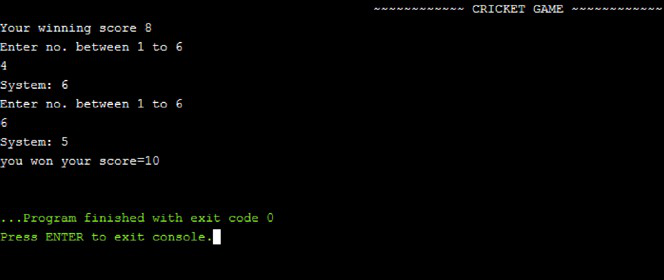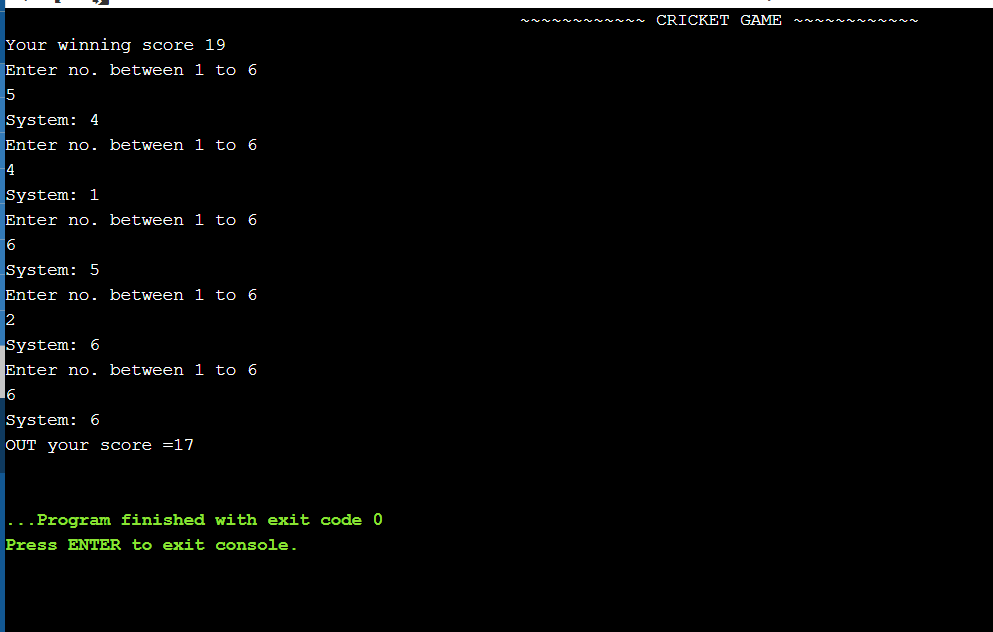GeeksforGeeks App
Open AppBrowser
Continue

# C/C++ program to implement the cricket game

In this article, the task is to create a 2- player cricket game where Player 1 is the user operating the program and Player 2 is the computer. In this game, the following series of steps are followed:

1. First, the program will generate a random number between 0 and 25. That number will be the score that Player 1 needs to make to win this match. Suppose the program-generated random number is 15, then this number will be the score that Player 1 needs to achieve.
2. The second step is a real match between Player 1(User) and Player 2(Computer).
3. The user will give input from numbers 1 to 6 using the keyboard. The system will then again generate a random number between 1 and 6. If the input of the user differs from the random number generated by the system, then it is a hit and the user will get the score as the same number that was given as input by the user. If the two numbers match, the user will be out and the final total score will be printed.
4. The total score scored by the user is the sum of all the numbers entered by the user. The score is calculated after each input by the user. Previous Input + Current Input of the user.

Example:

Random Number generated by System is 15. This is the winning score.

Now Player will enter number between 1 and 6.
Player: 2
System will now generate a random number between 1 and 6.
System: 1
The player is safe he can play further as both numbers are different.

Player will input a random number between 1 and 6.
Player: 3
System will now generate a random number between 1 and 6.
System: 4

This process keeps ongoing till same number is chosen by Player and System

Player: 6

System: 6

Now, as both numbers are same(player’s and system’s) then player is out.

Result: You lose, your total score is 5.

GAME OVER.

Below is the C++ program to implement the above approach:

## C++

 `// C++ program for the above approach``#include ``#include ``#include ``using` `namespace` `std;`` ` `// Driver Code``int` `main()``{``    ``int` `totalrun = 0;``    ``srand``(``time``(0));``    ``int` `i;`` ` `    ``// Generate a random number``    ``// and store in variable``    ``i = (``rand``() % 25) + 1;``    ``cout << ``"~~~~~~~~ CRICKET GAME ~~~"``         ``<< ``"~~~~~~~"` `<< endl;`` ` `    ``// Displaying the winning score``    ``cout << ``"Your winning score "``         ``<< i << ``"\n"``;`` ` `    ``// while loop for true condition``    ``while` `(1) {``        ``int` `player = 0;``        ``int` `a;`` ` `        ``if` `(totalrun > i) {``            ``cout << ``"you won your score="``                 ``<< totalrun << ``"\n"``;`` ` `            ``// To exit loop``            ``exit``(0);``        ``}``        ``else` `{`` ` `            ``// Generate random no. and``            ``// store in a variable``            ``a = (``rand``() % 6) + 1;``            ``cout << ``"Enter no. between "``                 ``<< ``"1 to 6"` `<< endl;`` ` `            ``// Taking input from user``            ``// to score runs``            ``cin >> player;`` ` `            ``// Checking if user's score``            ``// exceeds the winning score``            ``// Displaying random number``            ``// taken by system on screen``            ``cout << ``"System: "` `<< a << endl;`` ` `            ``// Check if number inserted``            ``// by user is the same random``            ``// number generated by system``            ``// inside loop``            ``if` `(player == a) {``                ``cout << ``"OUT your score ="``                     ``<< totalrun``                     ``<< endl;`` ` `                ``// To exit loop``                ``exit``(0);``            ``}`` ` `            ``// Storing total runs scored``            ``// by user``            ``else` `{``                ``totalrun = totalrun + player;``            ``}``        ``}``    ``}`` ` `    ``return` `0;``}`

Output:My Personal Notes arrow_drop_up